## On the Subject of Colorful Insanity

The madness can be even worse.

There’s a set of 35 buttons on the module.
You need to press the correct buttons in order to disarm the module.
First, you need to find 2 pairs of buttons:

1. 2 buttons that have the same pattern, but one has reversed colors.
2. 2 buttons that are exactly the same; they have the same pattern and colors.

With the pattern of the first pair, you can determine which patterns for the buttons are allowed for press.
On Table 1; locate where is your pattern, and the allowed patterns will be the ones that are directly adjacent to it. This means up/left/right/down.

Use Table 2 to determine the allowed colors, based on the colors of the second pair.
The color for the columns is the one with black color on Table 1.
The color for the rows is the other color.

### Color’s reference

• X: No color
• R: Red
• O: Orange
• Y: Yellow
• G: Green
• C: Cyan
• A: Azure
• B: Blue
• M: Magenta
• P: Purple
• Blank: Any color

You need to press the buttons that have both any of the allowed patterns AND any of the allowed colors.
If none of the buttons match, press the first and the second pair.

## Pattern table (Table 1)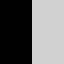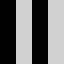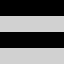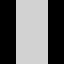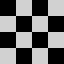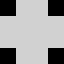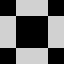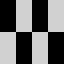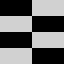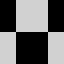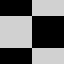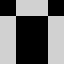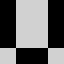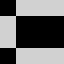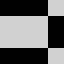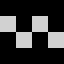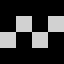## Color table (Table 2)

Red Orange Yellow Green Cyan Azure Blue Magenta Purple
Red X R R R R R R R R
Orange R X R R R R R R R
Yellow R R X R R R R R R
Green R R R X R R R R R
Cyan R R R R X R R R R
Azure R R R R R X R R R
Blue R R R R R R X R R
Magenta R R R R R R R X R
Purple R R R R R R R R X

See Color’s reference for the color letters.